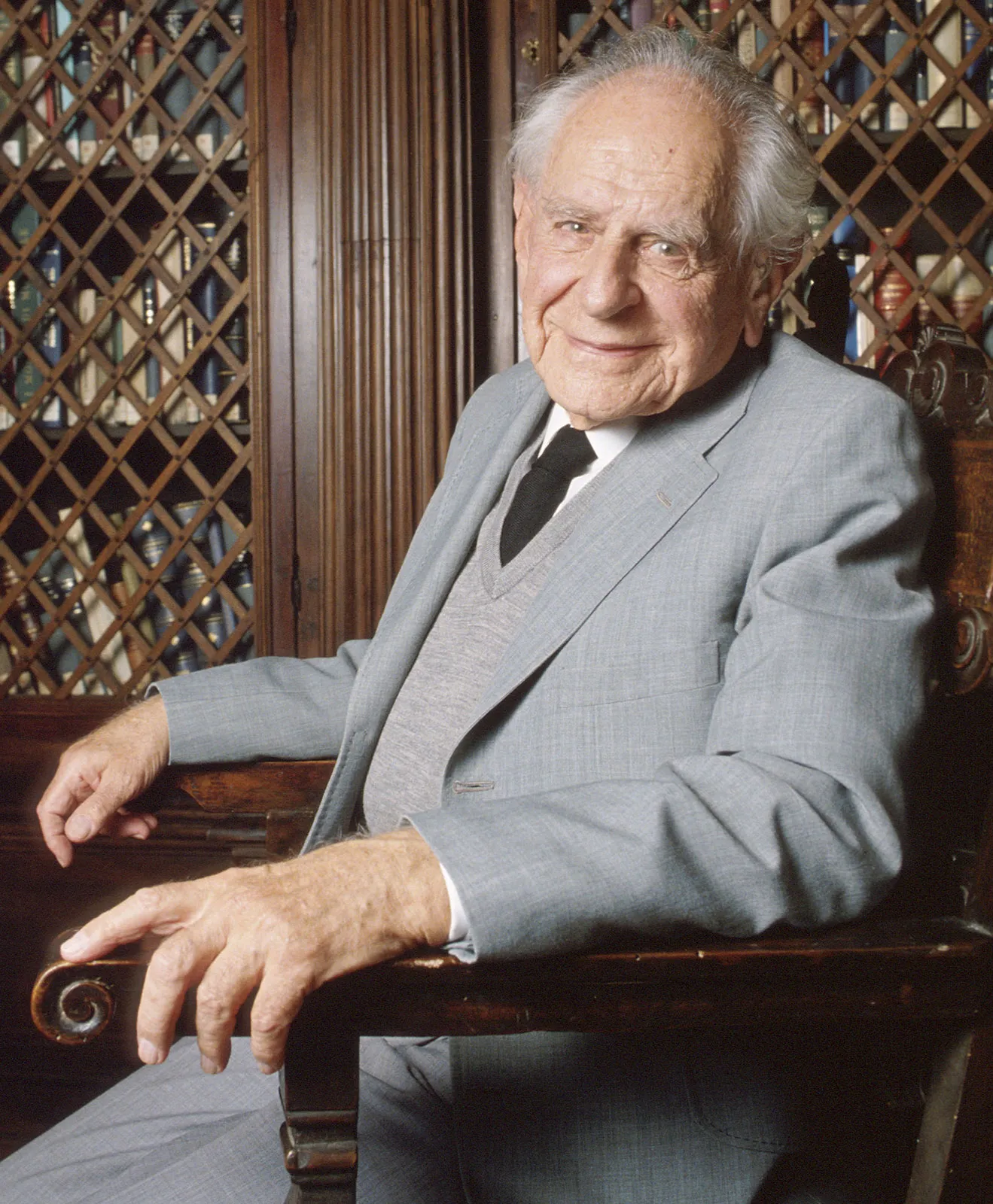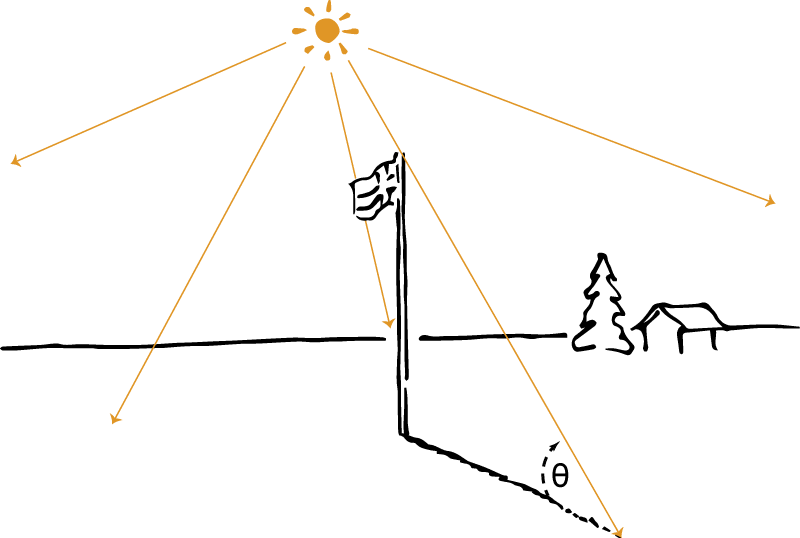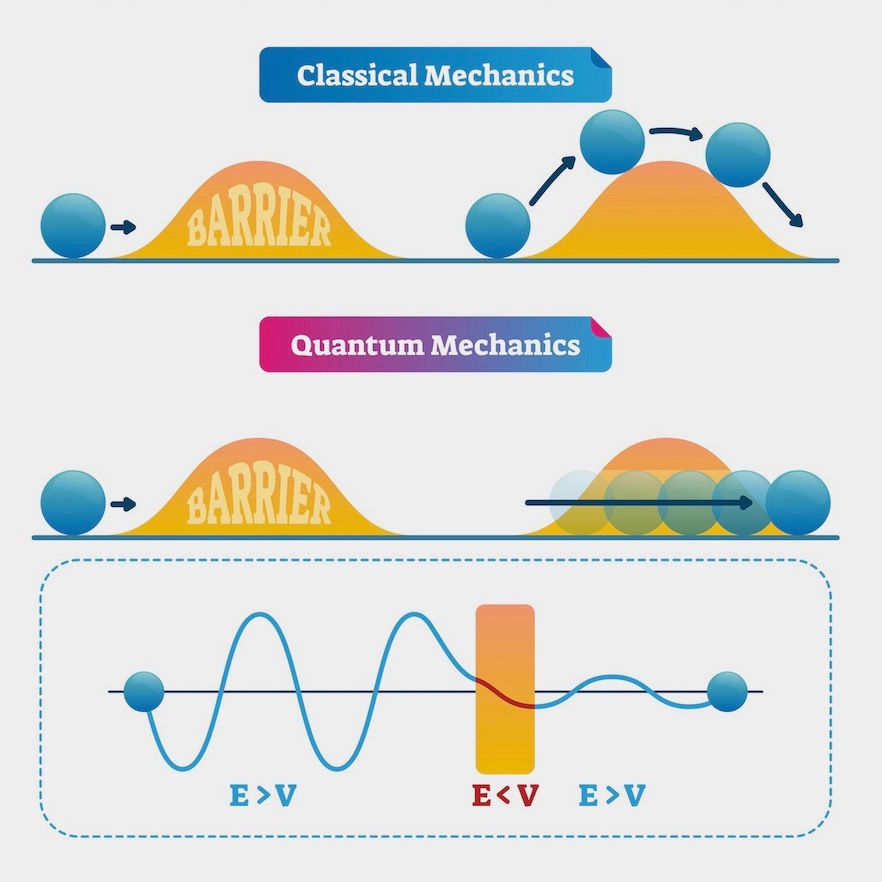top of page# Philosophy of Science 101: Models of Explanation

#### Foreword

The Philosophy of Science series explores both general questions about the nature of science and specific foundational issues related to the individual sciences. When applied to such subject areas, philosophy is particularly good at illuminating our general understanding of the sciences. This 101 series will investigate what kinds of serious—often unanswered—questions a philosophical approach to science exposes through its heuristic lens. This series, more specifically, will look at the ‘Scientific Realism’ debate throughout, which questions the very content of our best scientific theories and models.

Philosophy of Science 101 will be divided into the following chapters of content:

6. Philosophy of Science 101: Scientific Models

7. Philosophy of Science 101: Models of Explanation

8. Philosophy of Science 101: Laws of Nature

9. Philosophy of Science 101: Science and Social Context

#### Philosophy of Science 101: Models of Explanation

Previously, Philosophy of Science 101 explored scientific models. In particular, the previous article in this series investigated how models in science represent their target systems (i.e., ‘T’ —a particular part or aspect of the world that the given model is concerned with, such as the atom in Bohr’s (1913) model of the atom). The article considered the different types of models that represent T, and various accounts of representation which arise due to growing philosophical interest in this (crucial) part of scientific practice. Indeed, philosophical queries concerning representation—resulting from scientific modelling—are just one area of the philosophy of science interested in modelling. The series now turns to another area, namely models of scientific explanation.

Issues concerning scientific explanation have been a focus of philosophical attention from Pre-Socratic times through the modern period (Woodward and Ross, 2021). As this article will discuss, modern interest really begins with the development of the Deductive-Nomological (hereafter, ‘DN’) model. Thereafter, the philosophy of science not only sees well-known objections to the DN model in this area of study but also (i) various extensions of the DN model, and (ii) subsequent—notable—attempts to develop alternative models of explanation, oftentimes via statistical laws. Competing models of scientific explanation encounter interrelated issues which this article will both introduce and investigate in relation to the various conditions of adequacy. More specifically, this article will delve into the presupposition of the most recent philosophical discussion which has been that science sometimes provides explanations and that the task of a theory or model of scientific explanation is to characterise the structure of such explanations (Woodward and Ross, 2021).Figure 1. Bohr atomic model (Rogers, 2012).

Background: The Basic Idea

First of all, some brief background is needed. It is worth noting that science should answer so-called ‘why’ questions. For example, why does uranium 235 decay? Why did the solar eclipse occur? Answers to such questions take on the form of scientific explanations. Not all explanations, however, are scientific. Nor do all questions demand scientific explanations. Hence, the philosopher asks, what makes scientific explanations different? Before sussing out various proposed answers to these questions and the models available for scientific explanation, consider some terminology which will be used throughout the article:

Explanandum (Em): the ‘thing’ that has to be explained.

Explanans (Es): the ‘thing’ that does the explaining.

So, for example:

Explanandum: why does uranium 238 not undergo fission in a nuclear reactor?

Explanans: because of the large amount of energy needed.

(Cheifetz, Fraenkel, Galin, Lefort, Peter and Tarrago, 1970).

The DN Model of Explanation

Made famous by its proponents Carl Hempel and Paul Oppenheim (1948), the DN model of explanation—broadly speaking—is that one may explain something by subsuming it under general law. Hence ‘nomological’ (i.e., referring to laws). One may then show that Em is an instance of a general pattern. Consider the general structure of explanans and explanandum to constitute the explanation on the DN model (Hempel and Oppenheim, 1948):

L1, L2, … … … LK – Laws

A1, A2, … … … Am – Auxiliary Assumptions

B1, B2, … … … Bn – Boundary Conditions

–––––––––––––––––––––––––––

Implies, via logical deduction

E (conclusion).

The Es above (i.e., laws, auxiliary assumptions, and boundary conditions) imply Em, which provides the model of explanation.Figure 2. Carl Gustav "Peter" Hempel.

Consider a simple example of a solar eclipse to illustrate this general structure:

Laws: celestial laws of motion (i.e., both describing and showing how (a) planets move in elliptical orbits with the sun as a focus, (b) a planet covers the same area of space in the same amount of time no matter where it is in its orbit, and (c) a planet’s orbital period is proportional to the size of its orbit) (Russell, 1964)

Auxiliary assumptions: linear optics (i.e., the behaviour and properties of light)

Boundary conditions: the position of objects (i.e., the moon interposes itself between the Sun and the Earth, casting its shadow over the Earth) (Brown and Brown, 2017)

–––––––––––––––––––––––––––

Implies, via logical deduction

The solar eclipse occurrence x at t.

Most importantly, the conclusion is reached by logical deduction. Hence the ‘deductive-nomological’ model name, whereby an explanation is a deductively valid argument. Whether this is sufficient as a model of explanation, however, requires some further investigation into the conditions of adequacy for such a model of explanation.

Adequacy is the state of being sufficient for the purpose concerned (Fay and Moon, 1977) and is of utmost importance here, for an argument of this sort (i.e., the DN model) is an explanation if the following four conditions occur (Hempel and Oppenheim, 1948):

1. Em is a logical consequence of Es (i.e., the explanation is a valid deductive argument).

2. Es must contain at least one law, and this law must be used in the derivation of Em.

3. Es must have empirical content (i.e., it must at least in principle be empirically testable).

4. The sentences contained in Es must be true.

This is a deductive (i.e., structure of deductive) nomological (i.e., contains laws) explanation. On the DN model, explanations are arguments as such. The DN model can also, therefore, explain laws by appealing to more general laws (Cartwright, 1979) (as this article will turn to later). Moreover, Hempel and Oppenheim (1948) assume that the DN model applies equally well to both scientific explanation and scientific prediction. Arguably, it wouldn’t matter whether the DN model is used to show how theories explain certain events or how theories predict results. If the event has already occurred, it can be explained by the antecedent conditions and the theoretical laws. If the event has not occurred, it can also be predicted by the antecedent conditions and the theoretical laws (Pitt, 1988). Whether applied to explanation or prediction—and before considering statistical laws and probabilities—it is first worth considering the types of problems that the DN model encounters. There are two kinds of difficulties here, namely those against sufficiency and those against necessity. The first kind is concerned with arguments that satisfy all requirements but nevertheless fail to be explanations. Such arguments show that these requirements are not sufficient. On the other hand, the second kind is concerned with explanations that are considered to be real but do not satisfy the above requirements. Thus, such explanations are not necessary.Figure 3. Karl Popper, 1991. According to Popper, scientific trends stand in need of explanation.

Against Sufficiency

Another problem against sufficiency faced by the DN model comes from common causes (Pearl, 2000) (see figure 5), which the DN model does not rule out. The so-called ‘barometer example’ provides yet another famous example of a problem for the DN model here. Imagine there is a sharp drop in barometric pressure, resulting in the assurance that a storm is on the way (based on some kind of law that ties the two together). Indeed, one can predict the storm on the basis of barometric pressure. One does not want to claim that the storm is explained by the drop in barometric pressure, however, since both the storm and the drop in barometric pressure are caused by atmospheric conditions (Salmon, 2006). They have a common (i.e., the same) cause. Yet, as mentioned, the DN model does not rule this out. The laws given in the theory allow for predictions of all kinds. The problem is that those predictions are not the same thing as explanations: the storm does not explain the barometer reading, nor does the barometer reading explain the storm. Rather they are both explained by a third factor, the atmospheric conditions, which both cause and explain the two. The same issue around prediction versus explanation goes for the flagpole example too, for the shadow does not explain the height of the flagpole since it is instead the combination of the sun and the flagpole that causes the shadow (Hausman, 1998). Before moving on to the second kind of problem that the DN model encounters, it is worth noting that Hempel (1965) introduces the 'thesis of structural identity' to overcome the issues around prediction versus explanation. Hempel’s (1965) thesis supposes that (a) every adequate explanation is potentially a prediction, and (b) every adequate prediction is potentially an explanation. Hempel in fact defends (a) generally but acknowledges that (b) cannot be maintained in general (i.e., only in some cases). Overall, however, the ‘explanation and prediction’ problem against sufficiency remains and deserves attention elsewhere.Figure 4. The Flagpole problem (Roberts, 2014).

Against Necessity

As discussed, the DN model is in trouble due to a second kind of problem too. To reiterate, problems of this second kind faced by the DN model result from the explanations that are real (or are considered to be real) but do not satisfy the conditions of adequacy (i.e., that the explanandum is a logical consequence of the explanans, the explanans contain at least one law and contain empirical content, and the sentences contained in the explanans are true). Explanations here show that the requirements are not necessary. This article will briskly consider the problems which go against necessity before considering statistical laws to make up a model of explanation.

First, it is important to remember just what a significant role laws play in the nomological model. According to DN, one may explain something by subsuming it under general law. One may then show that Em is an instance of a general pattern, which is why a logical deductive argument constitutes an explanation. Further, the DN model can also—supposedly—therefore explain laws by appealing to more general laws. As Nancy Cartwright (1979) argues, however, regularities do not explain. The problem here for the DN model is that subsumption under a general law does not explain anything. Cartwright (1979) claims that what is needed are causes instead, which do have explanatory power. On this view, the DN model is simply wrong when it focuses on general regularities (if this is what laws consist of). Nothing is explained by laws, thus going against the DN model as a whole.Figure 5. A common cause structure. C occurs earlier than A and B, and C is a cause of A and B (Hitchcock and Rédei, 2021).

Another problem of this kind—which is somewhat similar to the problem Cartwright raises—comes from singular events. Singular events, as suggested by the name, are not regularly occurring events. Hence there are no regularly occurring laws (or patterns) involved here as such, therefore posing a threat to the DN model. A severe worldwide economic crisis like the stock market crash in 2008, for example. Though the 2008 financial crisis was notably the most serious financial crisis since the Great Depression (Barro and Ursúa, 2009), and there are of course other financial crises resulting from events that are ‘similar’ to that of the events occurring in 2007-2008, the stock market crash was nevertheless a singular event (with various Es explaining the Em, i.e., the crash) but which does not satisfy the DN model’s requirements to contain a law. Indeed, it is an example of an explanation that is considered to be real, showing that the DN model’s requirements are not necessary. Perhaps the use of statistical laws in a model of explanation is better?

Statistical Laws – The Inductive and Deductive Statistical Models of Explanation

Not all laws have the form (x) (Fx → Gx). Some laws, instead, have the form ‘some F are G’. More specifically, if x is an F then there is a probability (p) that x is also a G. These sorts of laws indeed play an important role in many of the sciences. The probability that a plutonium atom decays within an hour, for instance, or the probability of tunnelling in quantum physics (to name but just a few examples of many). Probabilities are undeniably essential in all the sciences and in the process of explaining. Probabilistic—statistical—laws are therefore used in various alternative models of explanation, namely the inductive statistical model (‘IS’) and the deductive statistical model (‘DS’).Figure 6. A diagrammatic representation of quantum tunnelling (Duffy, 2019).

According to Hempel (1965), an IS explanation is good or successful to the extent that its Es confer high probability on its Em outcome. The relation between Es and Em, unlike the DN model, is rather inductive. The difference is that IS explanations involve the subsumption of individual events under statistical laws (Woodward and Ross, 2021). Thus, the IS model explains a particular occurrence by subsuming it under a statistical law. This is unlike that of a DN or DS explanation which is deduced from the Es since it is a particular (individual) occurrence. Consider an example to illustrate how the IS model works (Woodward and Ross, 2021):

There is a 0.95 probability that patients with a streptococcus infection recover quickly after the administration of penicillin:

-----------------------------------------------------------------------------------------------------

-----------------------------------------------------------------------------------------------------

[0.95]

James recovers quickly.

So:

Statistical law: if x is an F then there is a probability, p, that x is also a G.

Particular condition: object a is an F.

------------------------------------------------------------------------------------------------

------------------------------------------------------------------------------------------------

[p]

Object a is a G.

Here, it was to be expected that object a is a G (i.e., that James recovers quickly, for instance) given certain explanatory facts and laws (i.e., the high probability of penicillin successfully treating streptococcus infections). Indeed, on the IS model, the value of p must be high, like in the streptococcus example. Crucially, not any probability explains: it must be a high (or practically certain) probability (Hempel and Oppenheim, 1948). Above all, the premises (i) make the conclusion highly probable, but (ii) do not imply the conclusion. The argument is not deductively valid like that of the DN model (hence ‘inductive’) (Woodward and Ross, 2021).